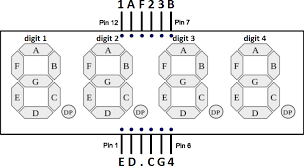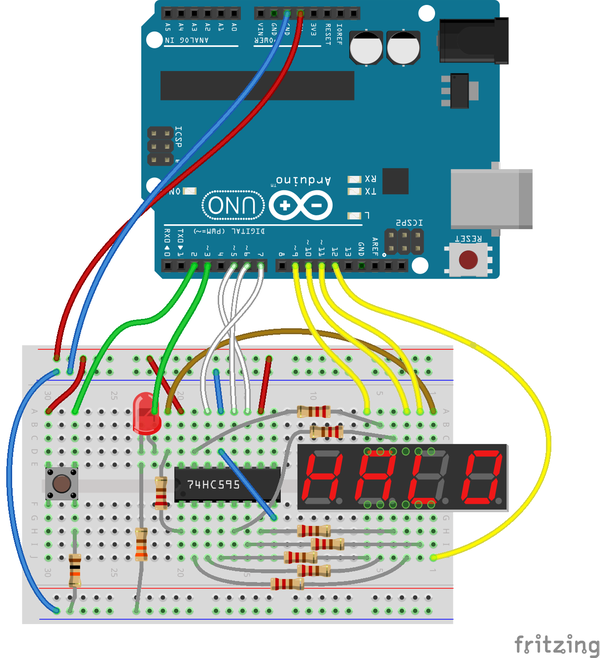A little, self contained demo of what you can do with Arduino.

Objective: challenge your friends response time, by pressing a button as fast as you can when an LED turns off. The time elapsed between the LED turning off and the button press is shown on a 4-digit display, in milliseconds.

Components:

• 1x Arduino Uno
• 1x Push-button switch
• 1x LED
• 1x 74HC595 (8 bit shift register)
• 1x HS410561K-32 (4 digits 7-segments display, common anodes)
• 8x 220 Ω resistors (current limiting the leds of the display)
• 1x 330 Ω resistor (current limiting the stand-alone led)
• 1x 10 kΩ resistor (button pull-down)

The shift register helps driving the 7 segments that compose each digit and the decimal point (totalling 8 leds for each digit), using only three Arduino pins. Eight bits are sent serially to the shift register, and the corresponding logical levels will be output in parallel on eight of its pins.

Since you can light up only one digit at a time, the microcontroller will have to continuously scan the display, activating in turn one of the four common anodes corresponding to the four digits.

#### Common anode, 4 digits display

The display has 12 pins: 4 pins correspond to the four digits (for example, the pin marked "1" - i.e. pin 12 - is the common anode of "digit 1"); the 8 remaining pins correspond to the seven segments (A to G) and to the decimal point.#### Wiring#### Sketch

The loop function is modeled around four states:

0 - idle: while waiting for a "player", the display shows seconds elapsed since power on; pressing the button enters next state

1 - the led is turned on for a random time interval between 4 and 9 seconds, and the display is not updated; the player is ready to catch the led turning off

2 - response time measurement: the display starts counting the milliseconds, until the player presses the button

3 - show result: the display shows the response time in milliseconds; a button press, or 60 seconds of inactivity, returns to the idle state

`const int digitPins = {9,10,11,12}; // display 4 common anodes (one pin per digit)const int clockPin = 5;    // 74HC595 Pin 11 (clock)const int latchPin = 6;    // 74HC595 Pin 12 (latch)const int dataPin = 7;     // 74HC595 Pin 14 (serial data in)const int ledPin = 3;const int buttonPin = 2;const byte digit =      // seven segment digits in bits{  B00111111, // 0  B00000110, // 1  B01011011, // 2  B01001111, // 3  B01100110, // 4  B01101101, // 5  B01111101, // 6  B00000111, // 7  B01111111, // 8  B01101111  // 9};int digitBuffer = {0,0,0,0};volatile byte btnEvent = 0;int s = 0; // state 0 = idle, 1 = ready random led time, 2 = measure resp time, 3 = show resultunsigned long tstart, resptime;unsigned long idlestart = 0;void setup() {  for(int i=0;i<4;i++)  {    pinMode(digitPins[i],OUTPUT);  }  pinMode(latchPin, OUTPUT);  pinMode(clockPin, OUTPUT);  pinMode(dataPin, OUTPUT);  pinMode(ledPin, OUTPUT);  pinMode(buttonPin, INPUT);    randomSeed(analogRead(0));  attachInterrupt(digitalPinToInterrupt(buttonPin), btnPressed, RISING);}// writes "value" on the 7-segments display,// with an optional decimal point at the specified positionvoid updateDisp(unsigned long value, byte decpos = 0) {  // load 4 digits in digitBuffer  digitBuffer = value % 10;      // units  digitBuffer = (value/10)%10;   // tens  digitBuffer = (value/100)%10;  // hundreds  digitBuffer = (value/1000)%10; // thousands  for (byte scandigit=0; scandigit<4; scandigit++) {    // set all common anodes (+) to LOW    for(byte j=0; j<4; j++) digitalWrite(digitPins[j], LOW);    digitalWrite(latchPin, LOW); // disable shift register output    // turn off all segments by setting HIGH all cathodes    shiftOut(dataPin, clockPin, MSBFIRST, B11111111);    digitalWrite(latchPin, HIGH); // enable shift register output    delayMicroseconds(100);        // set common anode of current digit to HIGH    digitalWrite(digitPins[scandigit], HIGH);    digitalWrite(latchPin, LOW); // disable shift register output    if ((decpos > 0) and ((decpos - scandigit) == 1))      // turn on decimal point near scandigit      shiftOut(dataPin, clockPin, MSBFIRST, ~(digit[digitBuffer[scandigit]] | B10000000));    else      shiftOut(dataPin, clockPin, MSBFIRST, ~digit[digitBuffer[scandigit]]);    digitalWrite(latchPin, HIGH); // enable shift register output    delay(1); // wait 1 ms, before turning on next digit  }}void loop() {  switch (s) {    case 0: // idle      digitalWrite(ledPin, LOW);      updateDisp(millis()/1000, (millis()/1000) % 4 + 1);      if (btnEvent) {        btnEvent = 0;        if (millis() > (idlestart + 1000)) { // debounce          s = 1;        }      }      break;    case 1: // led on for random time      updateDisp(0);      digitalWrite(ledPin, HIGH);      delay(4000 + random(5000));      if (btnEvent) {        btnEvent = 0;      }      digitalWrite(ledPin, LOW);      tstart = millis();      s = 2;      break;    case 2: // measure response time      updateDisp(millis() - tstart, 1);      if (btnEvent) {        btnEvent = 0;        resptime = millis() - tstart;        s = 3;      }      break;    case 3: // show result      digitalWrite(ledPin, HIGH);      updateDisp(resptime, 1);      if (btnEvent) {        btnEvent = 0;        if (millis() > (tstart + resptime + 1000)) { // debounce          idlestart = millis();          s = 0;        }      }      if (millis() > (tstart + 60000)) { // return to idle after 1 min        idlestart = millis();        s = 0;      }      break;          default:      s = 0;  }}void btnPressed() {  btnEvent = 1;}`

Current rating: 2

### New Comment

required

required (not published)

optional

required